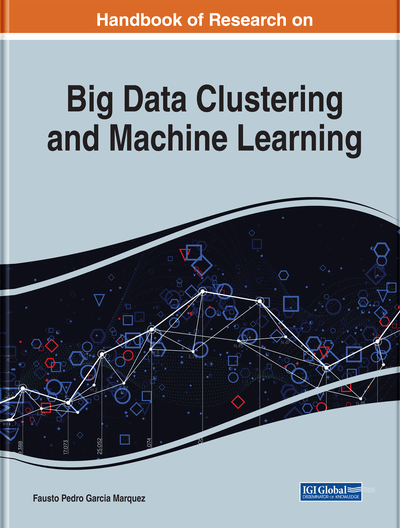# Enhanced Logistic Regression (ELR) Model for Big Data

Dhamodharavadhani S. (Periyar University, India) and Rathipriya R. (Periyar University, India)
DOI: 10.4018/978-1-7998-0106-1.ch008

## Abstract

Regression model is an important tool for modeling and analyzing data. In this chapter, the proposed model comprises three phases. The first phase concentrates on sampling techniques to get best sample for building the regression model. The second phase is to predict the residual of logistic regression (LR) model using time series analysis method: autoregressive. The third phase is to develop enhanced logistic regression (ELR) model by combining both LR model and residual prediction (RP) model. The empirical study is carried out to study the performance of the ELR model using large diabetic dataset. The results show that ELR model has a higher level of accuracy than the traditional logistic regression model.
Chapter Preview
Top

## Introduction

In the emerging data analytics for big data, regression analysis is one of them used in this research work. It mainly focuses the two class classification problems in the big data. The regression techniques are applicable only for minimal dataset having around some hundreds of records which is evident from the literature. So, the application of regression for big data is also a challenging task. The regression analysis is chosen because they entirely based on the variable dependency.

The data classification will be meaningful when that approach considers the relationships among the attributes. In that sense, the regression analysis is chosen in this research. This entire study deals with the logistic regression for big data having two class problem. Acquiring the knowledge by applying the regression to the entire large dataset is complex, so the sampling is one of the solutions to acquire the knowledge from the large dataset. The first phase data is sampled and then the regression analysis is performed on those samples. It concentrates on sampling techniques to get best sample for building the regression model. Second phase is to predict the residual of Logistic Regression (LR) model using time series analysis method- Autoregressive. Third phase is to develop Enhanced Logistic Regression (ELR) model by combining the both LR model and Residual Prediction (RP) Model. The empirical study is carried out to the study the performance of the ELR model using large diabetic dataset. The results show that ELR model has higher level of accuracy than the traditional Logistic Regression model

### Chapter Organization

The rest of the chapter is organized as follows: First section describes the literature study done for this research work. Second section deals with the methods and material used for the logistic regression analysis on big data. The experimental results of the proposed work are discussed in third section. Finally, summarizes this research work and suggests some ideas for future extension.

Top

## Review Of Literature

In the literature survey, the previous research contributions are studied. A lot of machine learning techniques are used for data analysis. They are discussed further in this chapter

(Strack, et al., 2014), An multivariate logistic regression is used to fit the relationship between the measurement of HbAlc early readmission while controlling for covariates such as demographics, severity and type of the dieses and type of admission. Results show that the measurement of HbAlc was performed frequently (18.4%) in the inpatient setting.

(Combes.C, Kadari.F, & Chaabane.S, 2014), A linear regression to identify the factors (variable) characterizing the length of stay (LOS) in Emergency department(ED) in- order to propose model to predict the length of stay.

(NM, T, P, & S, 2015), Using the predictive analysis algorithm in Hadoop and Map Reduce goal of their research deals with the study of diabetic treatment in HealthCare industry using big data analytics. The predictive analysis system of diabetic treatment is produce greats in healthcare. They mainly focused the patients in the rural area to generate proper treatment at low cast.

(Luo, 2016), The machine learning predictive model is using the electronic medical record dataset from practice fusion diabetes classification competition containing patient records from all 50 states in the united states. They explained the prediction result for 87.4% of patients who were correctly predicted by the model to have type 2 diagnoses with the next year.

(Carter & Potts, 2014), The poission regression and the negative binomial model for predicting length of stay were age, gender, consltant, discharge destination applying a negative binomial model to the variable was successful. The models can be successfully created to help improve resource planning and from which a simple decision support system can be produced to help patient explanation on length of stay.

(Ho, et al., 2016), An T Test, Chi square test and multivariate Logistic Regression analysis the variables in aciute stroke can predict in hospitality and help decision makes in clinical practice musing Nanogram. The nanogrames may help physicians in risk prediction in hospital mortality.

## Complete Chapter List

Search this Book:
Reset